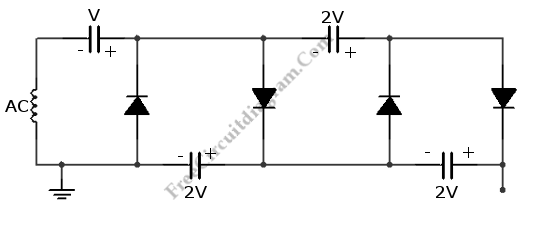# Voltage Multiplier with Diodes and CapacitorsThis is a voltage multiplier circuit. This circuit uses a doubler principle to multiply the voltage.  This circuit has some advantages like low current supply, attractive high voltage that is needed for photomultipliers and cathode-ray tubes. Besides that, this circuit is inexpensive. This principle is also used in the Cockroft-Walton accelerator for charged particles the Cockroft-Walton accelerator for charged particles since the obtain voltage is quite large. Here is the  schematic diagram of the circuit:This circuit can generate voltages of 2V, 4V and so on if the circuit is grounded like shown. It can produce V, 3V, 5V, and so forth if the circuit is grounded on other side. The problem of this circuit is an insulation. As the sections number is increased, the smaller current that is supplied. This circuit is easy to be implemented because this circuit uses silicon diodes. The component of this circuit should not resist the total voltage but only the stage voltage.